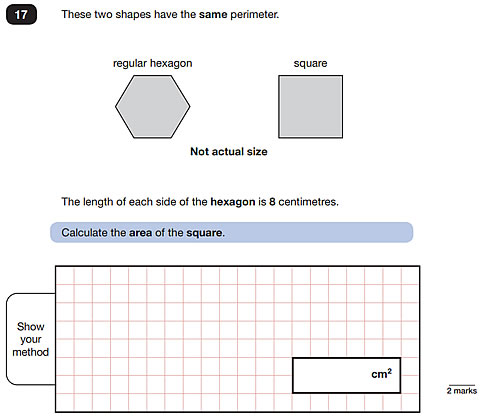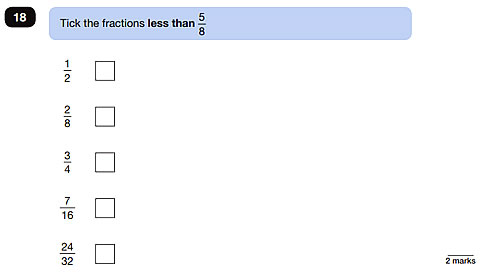• Article

The answer is only the beginning

A look at two of this year’s KS2 SATs questions, analysing the demands made on pupils and the mathematical thinking required• Published: 11/06/2019

The 2019 KS2 Maths SATs papers have been released and there has been a flurry of activity in the education wing of social media. Posts have appeared in almost equal measure loving and loathing the questions that thousands of 11-year-olds faced in May.

This article looks at two of this year’s questions. In both cases, we analyse the demands made on pupils and the mathematical thinking required to produce an answer.

In addition, as you go through each question, you’ll see some bold and hyperlinked text. In each case this will take you to a relevant section of our Mastery Professional Development Materials, which may prompt further thought about how lessons in these curriculum areas might be designed.

Question 17 from Paper 2For this question, pupils need to have flexibility of thinking. They have to work with a mixture of additive and multiplicative relationships in order to manage and apply the concepts of perimeter and area. These two ideas can be confusing to pupils, so being able to handle both in one question would demonstrate a good depth of understanding. [2.16 Multiplicative contexts: area and perimeter 1 and 2.30 Multiplicative contexts: area and perimeter 2, both available soon]

To gain their two marks, there were three steps for pupils to complete:

1. Calculate the perimeter of the hexagon from the length of one side
2. Calculate the length of the side of the square from its perimeter
3. Use the length of the side of the square to calculate its area.

So what structural understanding is required to complete these steps?

Perimeter, whilst we can calculate it using multiplication, is underpinned by repeated addition as we are working out the total length of the sides of the shape [2.2 Structures: multiplication representing equal groups]. Many pupils will associate the perimeter of the hexagon with 8 + 8 + 8 + 8 + 8 + 8, especially if they annotate the image with the side length. The strategy they then use to work out the answer of 48cm may vary from using a known times table fact (6 × 8) to using addition strategies to add the 8s together.

Once they get a perimeter of 48cm, they then have to apply the inverse to work out the length of the sides of the square. The structure they need here is partitive division (sharing) [2.6 Structures: quotitive and partitive division] as they are dividing 48 into 4 equal groups, each one the length of a side of the square. Again the known fact of 12 × 4 = 48 and its related division fact can be applied.

To calculate the area of the square, they have to remember a formula – area = length x width – as there are no grid lines on the shape. Pupils have to interpret the multiplication in their own way to work out the final answer of 12 × 12. This, it is hoped, will be a known fact.

There are two levels of thinking required here: working out the calculation from the structure of the problem, and also applying a structure to a remembered abstract formula. The numbers themselves are all within the expectation of their known times table facts, although they fall into the more challenging regions of these facts, drawing on knowledge of 4, 6, 8 and 12 times tables.

Question 18 from Paper 3This question involves fractions and inequality.

The procedural approach here would be to convert everything into 32nds and compare directly with but is there a more efficient and elegant way than that?

What would we want pupils to notice about the fractions presented in the question?

If pupils have had the chance to create and explore the generalisation that ‘for any fraction equal to a half, the numerator will be half of the denominator’ then they could use this to begin to make comparisons. [3.7 Finding equivalent fractions and simplifying fractions, available soon]

From this generalisation, we know that is greater than a half, so any fraction less than or equal to a half will be less than .

We can straight away tick and also and as they have numerators less than half their denominators.

That leaves and . Can we still use a half to help us?

is more than a half but is only more than a half. As , we can say that must be larger than .

That only leaves . An educated guess could be made that it is quite a few 32nds more than a half so it likely to be larger than but it could also be simplified to eighths or to quarters. Pupils might realise that is equivalent to and therefore we know already that it is larger.

There is a difference between these questions in the thinking required. The perimeter and area problem requires the same thinking from all pupils in order to solve it. There is no way around understanding the concepts of perimeter and area. If pupils are also fluent in times tables then the solution will be easier. Differences might arise in the way that they carry out the calculations required.

With the fraction problem, it is possible to apply a procedure to get the correct answer (converting all to a common denominator), but there are other ways of working which would indicate a greater depth and flexibility of thinking.

The bottom line is that ticks in the right boxes will gain pupils one or two marks, but finding out how the answer has been worked out gives insight into pupils’ depth of understanding of fractions.

Even with SATs, the answer is only the beginning in terms of assessing a pupil’s confidence and mathematical thinking.